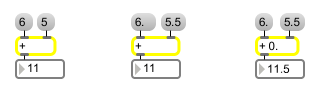# +

Add two numbers, output the result

## Description

+ takes any two given numbers, adds them, and then outputs the result.

## Arguments

Name Type Opt Description
initial-value int or float opt Sets the initial value, to be added to a number received in the left inlet. A float argument causes the numbers to be added as floats.

## Messages

 bang In left inlet: Performs the addition with the numbers currently stored. If there is no argument, + initially holds 0. int input [int] In left inlet: The number is added to the number in the right inlet, and the result is sent out the outlet. (inlet1) value-added-to [int] In right inlet: The number is stored for addition to a number received in the left inlet. float input [float] Converted to int, unless + has a float argument. set set-input [int] In left inlet: The word set, followed by a number, adds that number to the number in the right inlet but nothing is sent out. A subsequent bang sends out the result. The set message functions similarly for all the arithmetic operators, logical operators, and bitwise operators: +, -, *, /, %, <, <=, ==, !-, !/, !=, >=, >, &&, ||, &, |, <<, and >>. The number is used as the left operand, and the expression is evaluated, but the result is not sent out. list input [list] In left inlet: The first number is added to the second number, and the result is sent out the outlet.

## Output

float: Only if there is an argument with a decimal point.
int: The sum of the two numbers received in the inlets.

## ExamplesNormally adds ints... Floats are truncated before addition... unless there is a float argument